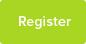Percentage Increases And Decreases

Notes on % increases and decreases

Date : 08/01/2016Subject : Maths

PERCENTAGE INCREASES AND DECREASES

With percentage increases and decreases there is typically a starting value, a percentage increase or decrease and an end or resulting value, with two of the three pieces of information provided and the third to be calculated.

We will start by looking at questions that give you the starting value and percentage increase or decrease and ask you to work out the resulting value.

Example

A house is worth £60,000 in 2010. Between 2010 and 2015 it increases in value by 40%. What is its value in 2015?

If the value in 2010 is £60,000 and we write this as 100%, then the finishing value is 140% (100% + 40%). So we need to work out what 140% of £60,000 is. This is exactly the same as

60,000 x 1.4 = 84,000

so the house is worth £84,000 in 2015.

Similar approach for a decrease. If the house decreases, rather than increases, in value by 40% then the finishing value is 60% (100% - 40%. Note the minus sign here, because the value has now fallen). This is exactly the same as

60,000 x 0.6 = 36,000

so the house is worth £36,000 in 2015.

Spend some time on the above. Given the starting value and a % increase of 15% what do you multiply the starting value by? What if the % increase is 10%? 5%? 1%? What do you multiply the starting value by if the value decreases by 15%? 10%? 5%? 1%?

B) Sometimes we get the end value and the percentage increase or decrease and need to work out the starting value.

Example

A house increases in value by 20% to £72,000. What was it worth before the rise?

If we write the original value as 100% then the final value , the £72,000, is 120%. So

72,000 = 120%.

Now work out what 1% is.

1% = 72,000/120

= 600

so

100% = 60,000

so the original value was £60,000.

[Note that we get exactly the same answer if we divide the £72,000 by 1.2. To see why, if we were given the starting value here ( £60,000) and the % increase, we would multiply the starting value by 1.2 to get the finishing value. Going the other way, we need to divide rather than multiply because division is the opposite of multiplication.]

Similar approach for a decrease. If the house is worth £72,000 now and it has decreased in value by 20%, then

72,000 = 80% (because 80 = 100 - 20).

Now work out what 1% is.

1% = 72,000/80

= 900

so

100% = 90,000

so the original value was £90,000.

Again, spend some time on the above. Given the finishing value and a % increase of 15% what do you do to get the starting value? What if the % increase is 10%? 5%? 1%? And what do you do if the value decreases by 15%? 10%? 5%? 1%?

C) The third possibility, the easiest, is where we are given both the starting and finishing values and asked to work out the percentage increase or decrease. See Q2 b) below.

Questions

Q1 A major high street store employs 3,655 people. This is a reduction of 14% compared to last year. How many people were employed by the store last year?

Q2 Write the missing numbers below (the ????? marks) to make each statement correct.

a) Houses cost £60,000 last year

They now cost ?????

This is a 25% increase

b) Houses cost £60,000 last year

They now cost £80,000

This is a ????? increase

c) Houses cost ????? last year

They now cost £80,000

This is a 25% increase

Q3 A car cost £8,000 last year. It is now worth 20% less than it cost. What is the car worth?

This resource was uploaded by: Rodelio